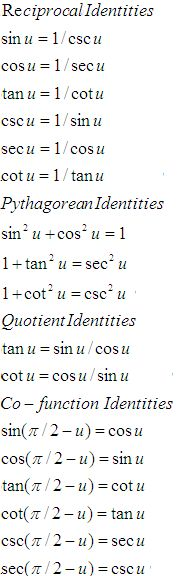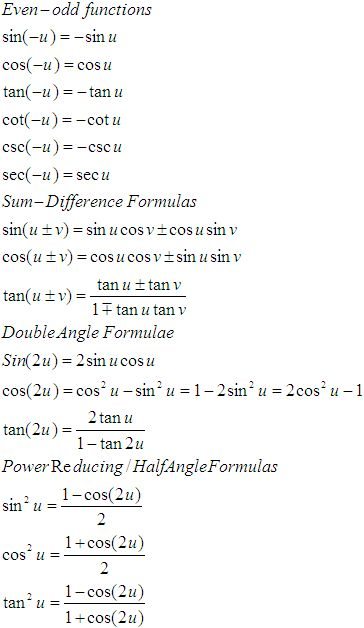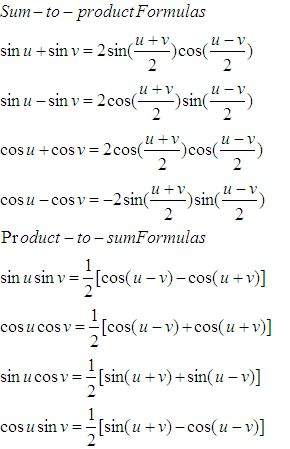Name: ___________________Date:___________________

 Email us to get an instant 20% discount on highly effective K-12 Math & English kwizNET Programs!

### High School Mathematics - 210.18 Trignometry Review

 Function 0o 30° 45° 60° 90° sin Ö0/2 Ö1/2 Ö2/2 Ö3/2 Ö4/2 cos Ö4/2 Ö3/2 Ö2/2 Ö1/2 0 tan 0 Ö3/3 1 Ö3 undefined sec 1 2Ö3/3 Ö2 2 undefined csc undefined 2 Ö2 2Ö3/3 1 cot undefined Ö3 1 Ö3/3 0

 sin cos tan 0 0 1 0 90 1 0 infinity 180 0 -1 0 270 -1 0 infinityFunctions of angles in all quadrants in terms of those in quadrant 1
 -A 900 ± A P/2 ± A 1800 ± A P ± A 2700 ± A 3P/2 ± A 3600 ± A 2P ± A sin -sinA cos A ±sin A -cosA ±sin A cos cos A ±sin A -cos A ±sin A cos A tan -tan A ±cot A ± tan A ±cot A ±tan A csc -csc A sec A ± csc A -sec A ±csc A sec sec A ± csc A -sec A ±csc A sec A cot -cot A ± tan A ± cot A ±tan A ± cot A

 Q 1: If the shadow of a tower is 30 metres, when the sun's altitude is 30o, what is the length of the shadow when the sun's altitude is 60o?Answer: Q 2: Find the angle in radian through which a pendulum swings if its length is 75 cm and the tip describes an arc of length 10 cm2/154/153/15 Q 3: The angular elevation of a tower from a point is 30 degrees at a point in a horizontal line to the foot of the tower and 100 metres near it is 60 degrees, find the distance of the first point from the tower. 100 metres150 metres86.6 metres Q 4: Solve the triangle ABC given that A = 67o, b = 3 cms, c = 2 cms.a = 5 cm, B = 70o19l, C = 39o 41la = 2 cm, B = 73oC = 39o 41la = 2.88 cm, B = 73o19l, C = 39o 41l Q 5: A person standing on the bank of a river, observes that the angle subtended by a tree on the opposite bank is 60 degrees, when he retires 40 metres from the bank, he finds the angle to be 30 degrees. Find the height of the tree.20 metres34 metres64 metres Q 6: In triangle ABC b = 4, c = 6, B = 30o, find sin C.3/44/31/2 Q 7: Find the degree measure of the angle subtended at the centre of a circle of diamter 200 cm by an arc of length 22cm.45o12o36l33o Q 8: In triangle ABC, a = 50, c = 60, b = 100 degrees, find b.8084.585 Question 9: This question is available to subscribers only! Question 10: This question is available to subscribers only!